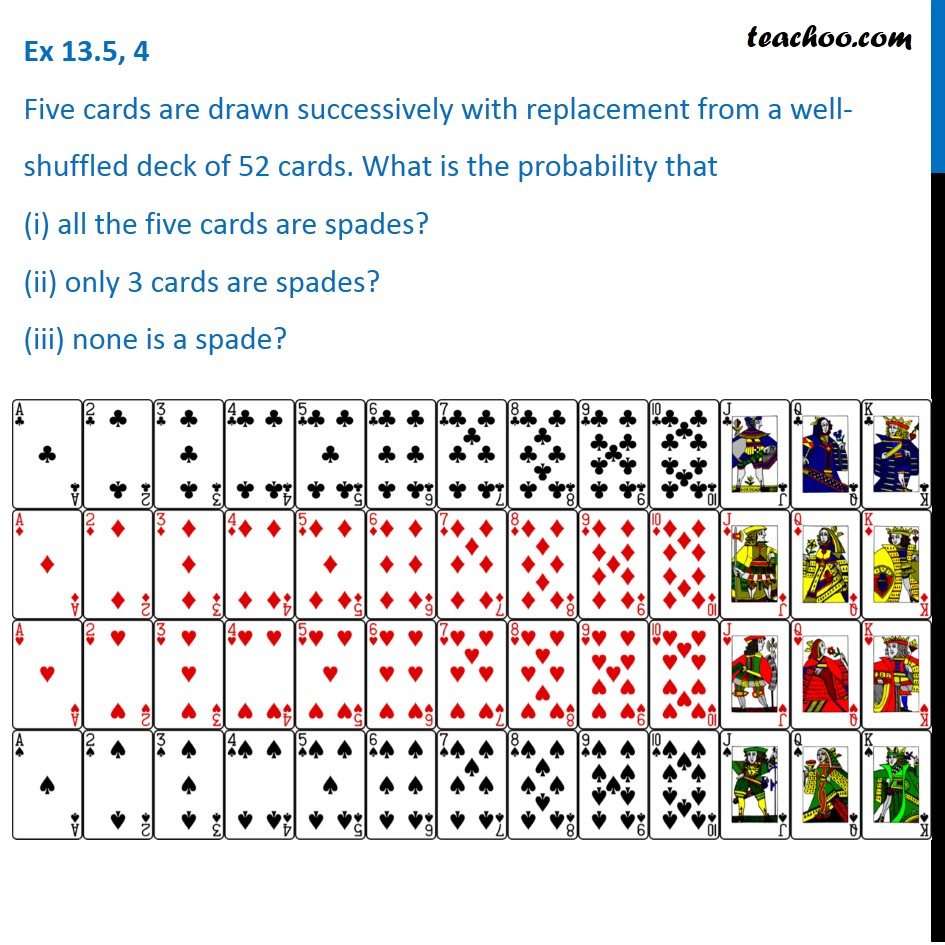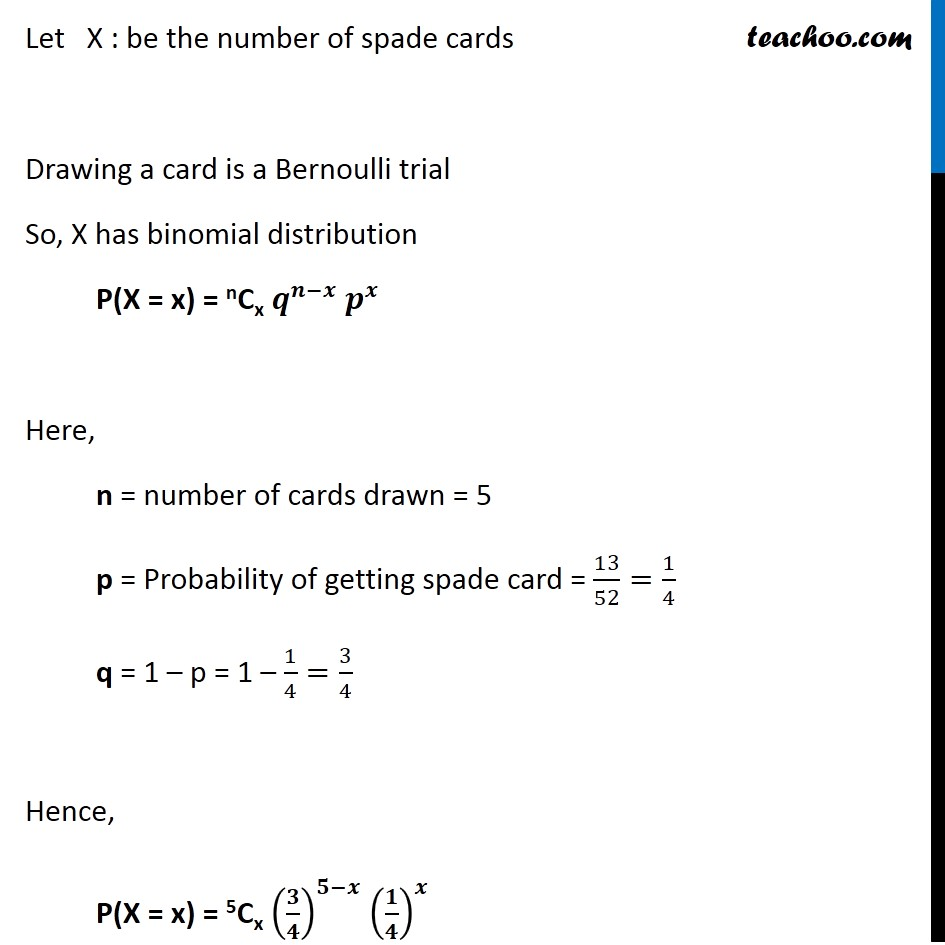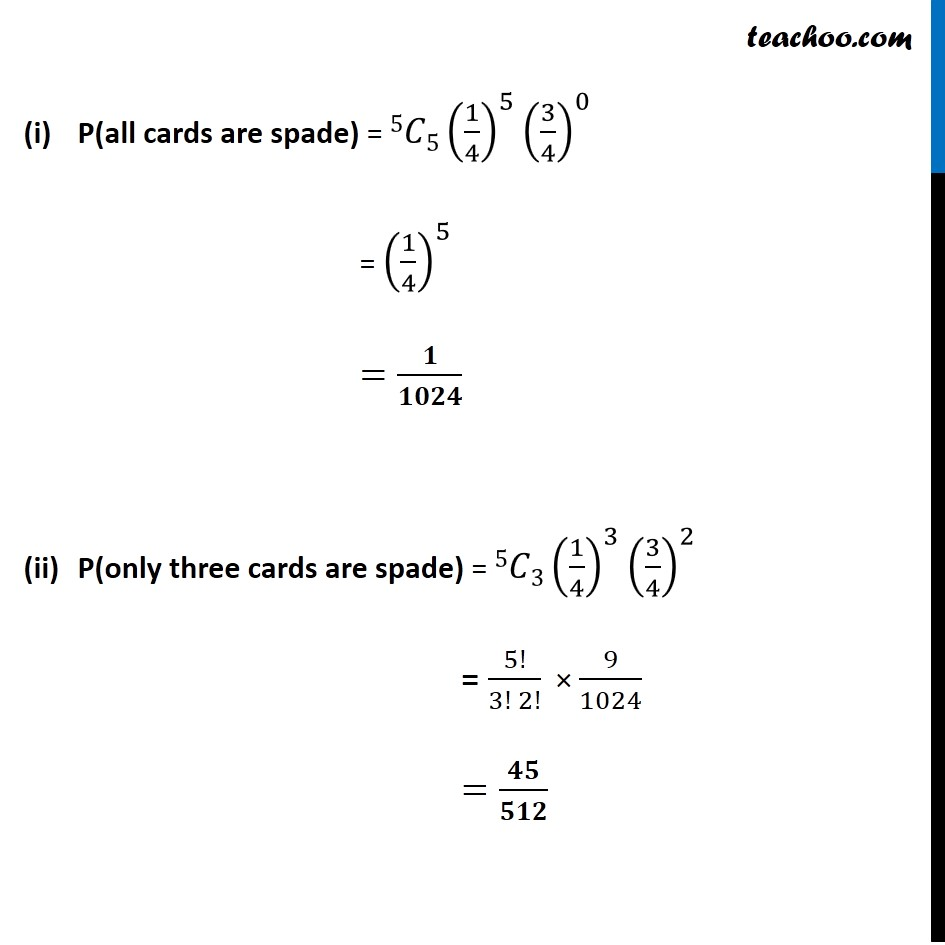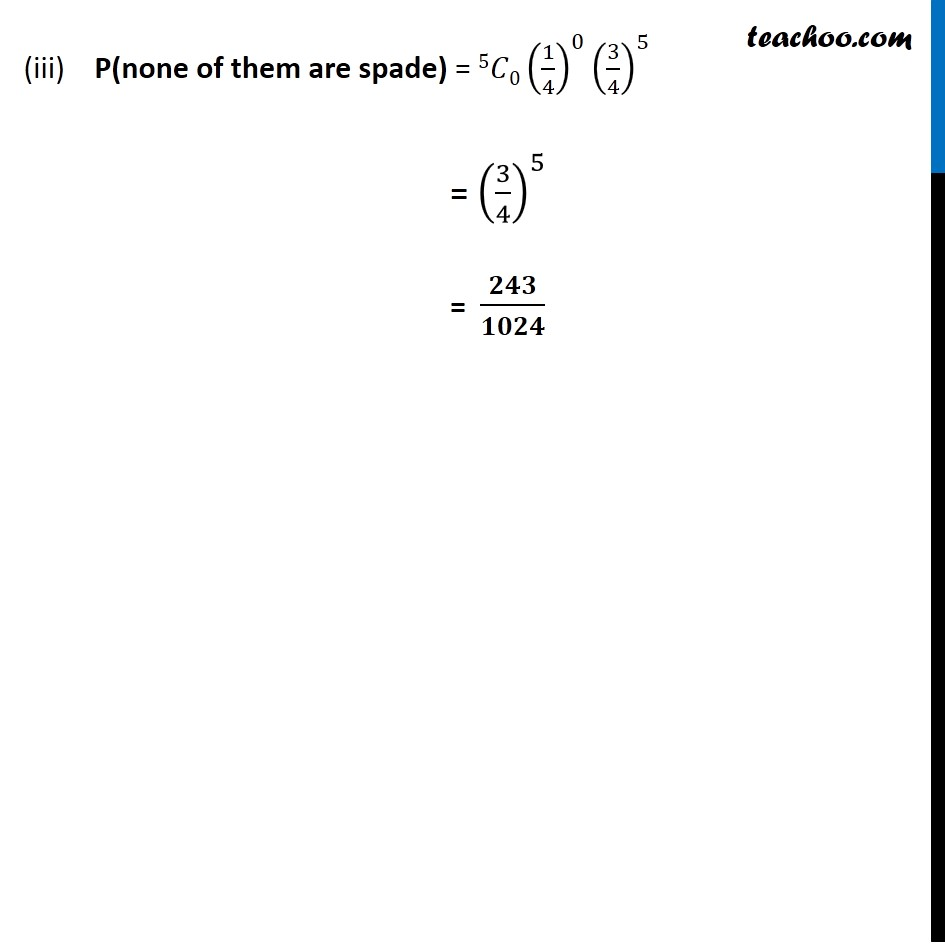Bernoulli Trial

Chapter 13 Class 12 Probability
Serial order wiseLearn in your speed, with individual attention - Teachoo Maths 1-on-1 Class

### Transcript

Question 4 Five cards are drawn successively with replacement from a well-shuffled deck of 52 cards. What is the probability that (i) all the five cards are spades? (ii) only 3 cards are spades? (iii) none is a spade?Let X : be the number of spade cards Drawing a card is a Bernoulli trial So, X has binomial distribution P(X = x) = nCx 𝒒^(𝒏−𝒙) 𝒑^𝒙 Here, n = number of cards drawn = 5 p = Probability of getting spade card = 13/52=1/4 q = 1 – p = 1 – 1/4=3/4 Hence, P(X = x) = 5Cx (𝟑/𝟒)^(𝟓−𝒙) (𝟏/𝟒)^𝒙 P(all cards are spade) = 5𝐶5(1/4)^5 (3/4)^0 = (1/4)^5 =𝟏/𝟏𝟎𝟐𝟒 P(only three cards are spade) = 5𝐶3(1/4)^3 (3/4)^2 = 5!/(3! 2!) × 9/1024 =𝟒𝟓/𝟓𝟏𝟐 (iii) P(none of them are spade) = 5𝐶0(1/4)^0 (3/4)^5 = (3/4)^5 = 𝟐𝟒𝟑/𝟏𝟎𝟐𝟒